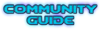Archive of the old editing forum
Forum rules
Before asking on how to use a ZDoom feature, read the ZDoom wiki first. This forum is archived - please use this set of forums to ask new questions.NashPosts: 17340
Joined: Mon Oct 27, 2003 12:07 am
Location: Kuala Lumpur, Malaysia

Code: Select all

``````
double a = 42.f;

double b = 360. / 2;

``````
1) When to use or not use the period after the number, if there is no fractional part?
2) What does it even mean?
3) When are you supposed to use f and not use f?
dpJudasPosts: 2921
Joined: Sat May 28, 2016 1:01 pm

### Re: About the double type

Not sure if this is the correct answer for ZScript, but for C++ the .f is shorthand for float, while just the dot is shorthand for double.

If you are storing something in a double it is a bad idea to use .f because that may make the calculations a little less accurate. In your example, though, it makes no difference because a float can fully hold the number 42 without any precision loss.

Generally for ZScript I would always use doubles, unless you have a very specific reason to use floats.Graf ZahlPosts: 48514
Joined: Sat Jul 19, 2003 10:19 am
Location: Germany

### Re: About the double type

The '.f' part really has no meaning in ZScript because even if present, it's still using strtod to convert the number into a double. And if you declare a local variable 'float' it will also be made a double implicitly. Single precision floats can only be used as struct, class or array members.
dpJudasPosts: 2921
Joined: Sat May 28, 2016 1:01 pm

### Re: About the double type

Nash wrote:1) When to use or not use the period after the number, if there is no fractional part?
Forgot to answer that one. When you add the period that number becomes a double, while if you leave it out the number is an integer. Here's some examples:

Code: Select all

``````int a = 5 / 2; // result is 2, because its an integer division
double b = 5 / 2; // result is still 2, because it is an integer division, then converted to a double
double c = 5. / 2; // result is 2.5, because a double divided by an integer is a floating point division
double d = 5 / 2.; // result is 2.5, same reason
double e = 5 / 2 + 5. / 2; // result is 4.5 - first part is an integer division, second part is a float
``````
Which to use: depends if you want to calculate with integer or floating point arithmetics.NashPosts: 17340
Joined: Mon Oct 27, 2003 12:07 am
Location: Kuala Lumpur, Malaysia

### Re: About the double type

Thanks! So when I want to specifically make the result have floating point precision, I must enforce it by adding the period. :D